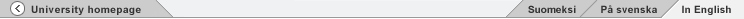581257 Information Retrieval Methods

Weekly exercises

Find the exercises in the course book. The numbers of the exercises come from the online version for printing (pdf) which are the same as in the printed edition.

1. Week 1 (10 Sep)
1. Exercise 1.2
2. Exercise 1.7
3. Exercise 1.13
4. Exercise 2.1
5. Exercises 3.1, 3.2, 3.3
6. Exercise 3.5

2. Week 2 (17 Sep)
1. Exercises 6.5, 6.6
2. Exercise 6.10
3. Exercises 6.15, 6.16
4. Exercise 6.17
5. Exercise 7.2
6. Exercise 7.7

3. Week 3 (24 Sep)
1. Exercise 8.1
2. Exercise 8.8
3. Exercise 8.10
4. Exercise 9.4
5. Exercise 9.5
6. Exercise 9.6

4. Week 4 (1 Oct)
1. Exercise 11.2
2. Exercise 11.4
3. Exercise 12.1
4. Exercise 12.2
5. Exercises 12.3, 12.4
6. Exercise 12.6

5. Week 5 (8 Oct)
1. Exercises 19.3, 19.4
2. Exercise 19.5
3. Exercise 19.7
4. Exercise 19.8
5. Exercise 20.1
6. Exercise 20.4

There is class this week. Submit your solutions by email or paper (via the custodians first floor) to Juha Makkonen.Search department or university site: Feedback | Faculty homepage | University homepage